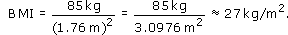Starting with maths: Patterns and formulas

Start this free course now. Just create an account and sign in. Enrol and complete the course for a free statement of participation or digital badge if available.

Free course

# 3.3 Maths in healthcare

The body mass index (BMI) is sometimes used to help determine whether an adult is under- or overweight. It is calculated as follows: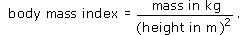Although care needs to be taken in interpreting the results (for example, the formula isn't appropriate for children, old people or those with a very muscular physique!), a BMI of less than 20 suggests the person is underweight and a BMI of over 25 suggests the person is overweight.

In this formula, the units have been included in the expression on the right-hand side of the equals sign. It is important to change any measurements into these units before you substitute the values into the formula. (No units have been included for the BMI on the left-hand side, in line with current health-care practice. However, strictly the unit for the BMI is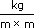which is the same as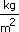or ‘kilograms per square metre’.)

## Activity 5: Body mass index

If an adult man is 176 cm tall and has a mass of 85 kg, calculate his BMI and decide whether he is overweight.

### Discussion

The formula needs the mass in kilograms and the height in metres. So convert the height into metres first.

Since there are 100 cm in a metre, 176 cm = 176 ÷ 100 m = 1.76 m.

Substituting the height and the mass into the formula for the BMI gives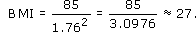Since his body mass index is over 25, the man is probably overweight.

Can you work out what weight he should be?

Note that, if you study science courses, scientists include the units throughout the calculation writing the solution like this: